# Catch up on the latest AI articles# Cal-QL: Offline Reinforcement Learning Specialized For Prior Learning, For Efficient Online Fine Tuning

3 main points
✔️ Experimental investigation of offline reinforcement learning + online fine tuning challenges
✔️ Proposes an offline reinforcement learning method for pre-training, Calibrated Q-Learning (Cal-QL), which improves on existing methods based on discussion of the investigation results
✔️ Offline reinforcement Learning + Online Fine Tuning scenarios, outperforms existing methods

writtenby Mitsuhiko NakamotoYuexiang ZhaiAnikait SinghMax Sobol MarkYi MaChelsea FinnAviral KumarSergey Levine
(Submitted on 9 Mar 2023 (v1), last revised 20 Jun 2023 (this version, v2))
Comments: project page: this https URL

Subjects: Machine Learning (cs.LG); Artificial Intelligence (cs.AI)code：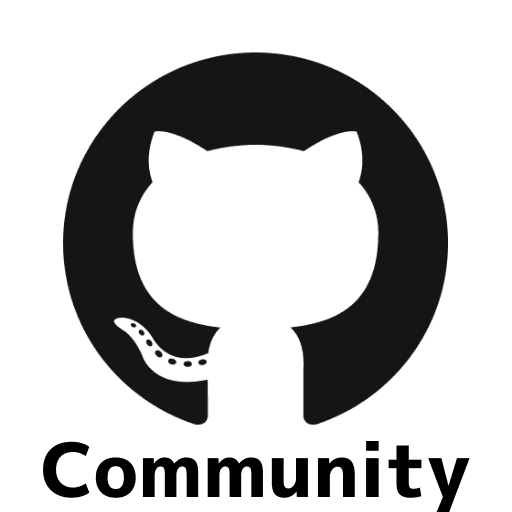The images used in this article are from the paper, the introductory slides, or were created based on them.

## Introduction

The framework of fine-tuning models pre-trained by large-scale data for individual applications has shown its effectiveness in real-world applications of deep learning techniques. In image recognition and natural language processing, large-scale image models (e.g. Imagen) and large-scale language models (e.g. ChatGPT) have been developed not only for industrial development but also for everyday convenience.

In response to such a trend, a framework for pre-training and fine-tuning strategies with minimal additional interaction in the target environment has been actively studied in reinforcement learning in recent years, using off-line reinforcement learning techniques to learn strategies from static data.
Once such techniques are established, it is expected that deep learning techniques will be further utilized in fields such as automatic driving and medical robotics, where the number of interactions with the environment is limited due to cost and risk.

This study focuses on off-line reinforcement learning as prior learning. As indicated in the first main point, this study consists of the following three parts (1) Experiments are conducted to investigate the performance and challenges of using existing off-line reinforcement learning methods for pre-training. (2) We propose a simple improvement to overcome the issues found in the study. (3) Experimental verification of the effectiveness of the proposed method as a pre-training method. In this article, after explaining the basics of off-line reinforcement learning, the above three points are explained step by step.

## Offline Reinforcement Learning

In offline reinforcement learning, we aim to learn an optimal strategy without additional interaction with the environment based on the interaction data $D = \{s_i, a_i, r_i, s'_i\}_{i=1}^{n}$ collected from some measure ($\pi_\beta(a|s)$). Since we cannot interact with the environment, we cannot collect additional state-action pairs that are not present in the data. Therefore, there is no way to correct for overestimation of Q-values of state-action pairs that do not exist in the data, for example. Such problems caused by state actions that do not exist in the data are called Out-Of-Distribution (OOD) problems, and are the biggest challenge in offline reinforcement learning. Existing countermeasures to the OOD problem can be classified into two main categories: conservative methods and methods that impose constraints on the measures. In this paper, we propose a method based on the conservative method, which is explained in more detail.

### Conservative approach

In order to prevent the overestimation of the Q-value shown earlier, this method estimates the Q-value by discounting it so that the estimated Q-value becomes the lower boundary of the true value function.
This method is conservative in the sense that it does not overestimate the Q-value. A representative conservative method is Conservative Q-Learning (CQL), which is also discussed in this study. The difference between CQL and the usual method using the Q-function is explained below. We assume that the Q-function is parameterized by the parameter $\theta$.

・Conventional method

$$\min_{\theta} \frac{1}{2} (Q_{\theta}(s, a) - \mathrm{B}^{\pi}Q(s, a))^2$$

where $\mathrm{B}^{\pi}$ is the expected Bellman operator of measure ($\pi$), and the objective function is an estimation problem of the value function of measure ($\pi$).

・CQL

$$\min_{\theta} \underbrace{\alpha(\mathbb{E}_{(s, a)\sim \pi}[Q_{\theta(s, a)}] - \mathbb{E}_{(s, a)\sim D}[Q_{\theta(s, a)}])}_{penalty term} + \ frac{1}{2} (Q_{\theta}(s, a) - \mathrm{B}^{\pi}Q(s, a))^2$$

Here, the penalty term constrains the expected value of the Q-function of the measure ($\pi$) not to depart from the expected value of the Q-function for the data distribution. In other words, we require the Q function to be conservative against overestimation of state actions that are not in the data . Theorem 3.2 of the CQL paper proves that the expectation of the Q-function estimated by the above objective function with the policy $\pi$ is a lower bound of the true $\pi$ value function. See the reference if you are interested.

### A method of constraining the measure $\pi$

In this method, optimization is performed with constraints to ensure that the policy to be learned ($\pi$) does not deviate significantly from the policy that generated the data ($\pi_\beta$). Constraints can be introduced in various ways, the most common of which is to use KL-divergence, which measures the distance between distributions. IQL, AWAC, and TD3-BC are examples of methods that place constraints on measures.

## Survey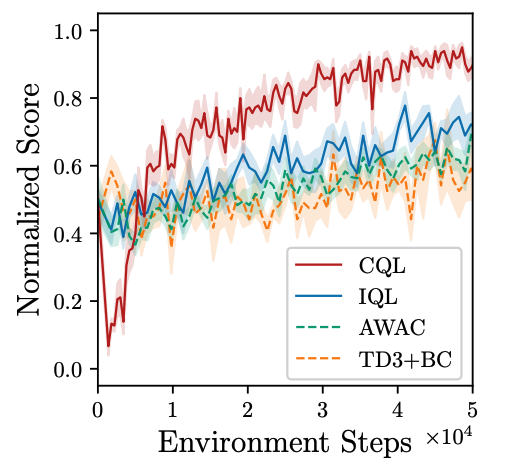Figure 1: Results of fine tuning of measures pre-trained with existing offline reinforcement learning.

### Setting

We compare the performance of pre-training plus fine tuning using existing off-line reinforcement learning methods, CQL, IQL, AWAC, and TD3-BC. The benchmark used for the comparison is a manipulation task that picks up an object from an image input and moves it to a specific location.

### Result

The following two points can be observed from Figure 1.

• Constraint-based methods, IQL, AWAC, and TD3-BC are all slow in learning.
• CQL, a conservative method, showed a significant performance degradation in the early stages of learning, although the final learning results were good.

### Consideration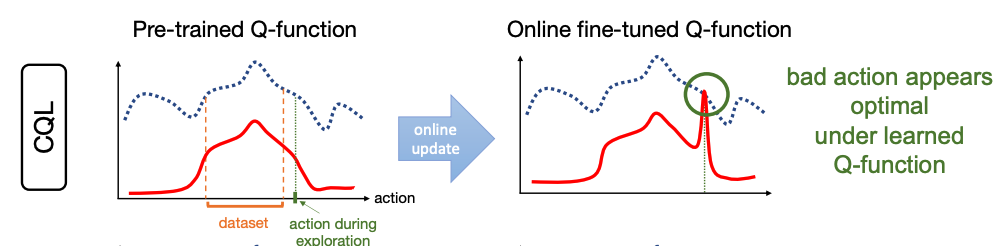Figure 2. The bluedotted line is the true Q value and the redsolid line is the estimated Q function.

In this paper, we consider CQL with its high final performance as a promising pre-training method, and examine the causes of CQL's issue of poor performance in the early fine-tune stage.

In the conservative approach, the penalty term results in a smaller overall estimate of the Q value. When choosing actions by the Q function, even if the value scale is wrong, the optimal action can be chosen during inference as long as the learning of the small and large Q-value relationships among actions is sufficient.
However, once online fine tuning starts, the Q function returns to the actual value scale [Figure 2]. Using a Q function that is underestimated compared to the actual Q function as the initial value, the value of the suboptimal action taken online is estimated to be relatively high in the early stage of learning, simply because it is back on the actual scale . As a result , they consider that the strategy learns to target suboptimal actions, resulting in unlearning.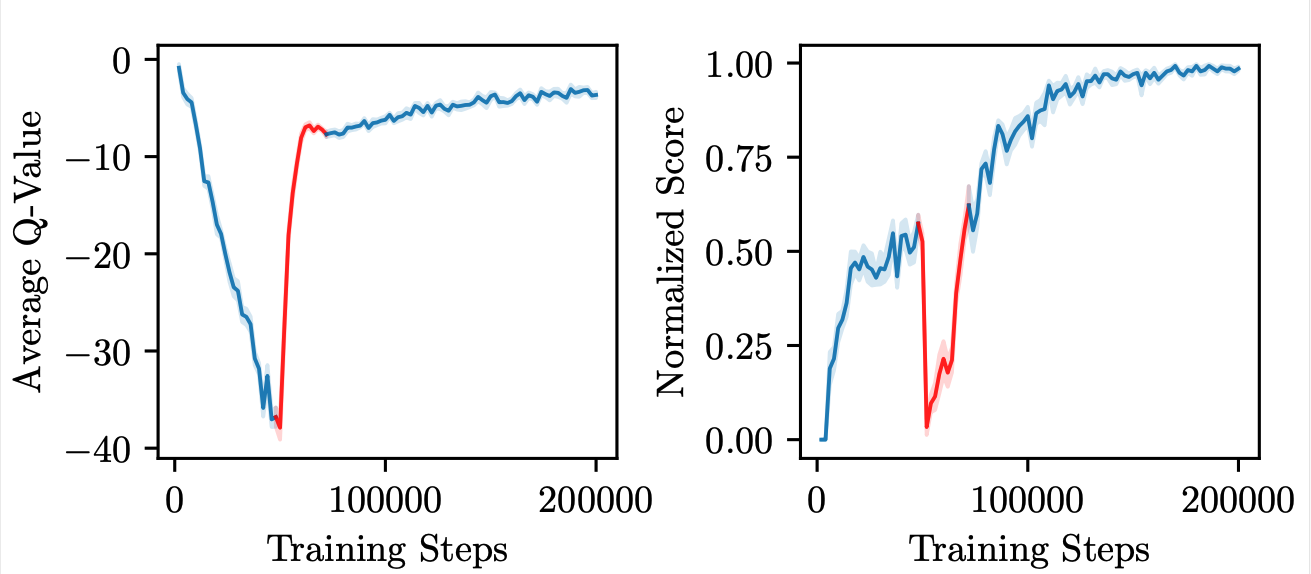Figure 3. Mean Q-function and performance through pre-training and fine tuning. Fine tuning begins at the 50k step.

As evidence to support this hypothesis, they plot the mean Q-values and performance estimated by CQL through pre-training and fine tuning [Figure 3]. Fine tuning starts at the 50k step, and it can be observed that a sharp increase in the mean Q-value and a sharp drop in performance occur around this step.

## Technique

In the experiments described above, we confirmed a phenomenon called "unlearn" in which the scale of the Q-function becomes smaller than the actual one in the pre-training using conservative methods, and the learning becomes unstable because the non-optimal actions have relatively high values in the early stage of the online fine-tuning process. In this paper, we propose a more efficient pre-training method to prevent this phenomenon by estimating the Q-function so that it is conservative with respect to the actual Q-function, but higher than the Q-function of the suboptimal measures. The above Q-function that "takes a higher value than the Q-function of the suboptimal measure" is defined as "Calibrated" as follows

### Definition 4.1 Calibration

The Q-function $Q^{\pi}_\theta$ estimated for a measure is calibrated for the reference measure $\mu$ if for any state $s$, $\mathbb{E}_{a\sim \pi}[Q^{\pi}_\theta(s, a)]\geq \mathbb{E}_{a\ sim \mu}[Q^{\mu}(s, a)]$ is true.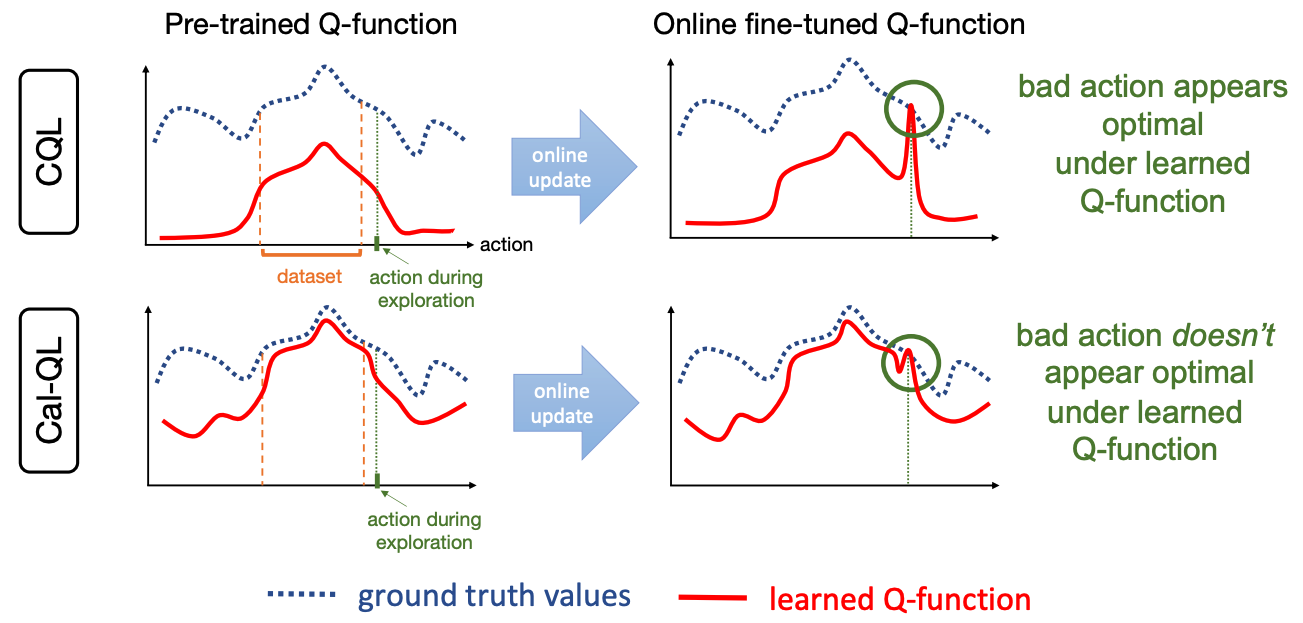Figure 4. Schematic diagram of the difference in the initial behavior of fine tuning between CQL and Cal-QL. In CQL, as explained earlier, "unlearn" occurs when the Q-value of a suboptimal action becomes excessively high due to the return to scale. On the other hand, using the algorithm for calibrating the Q function (Cal-QL), the Q value is guaranteed to be higher than the value of the suboptimal measure, so that the suboptimal behavior is not over-estimated.

If the estimated Q function is calibrated for all suboptimal measures $\mu$, the Q function is guaranteed to upper bound the measures with suboptimal behavior. Therefore, the Q-value of the suboptimal action will never exceed the maximum Q-value estimated by pre-training, thus preventing "unlearn". [Figure 4].

### Cal-QL

As a practical way to realize calibration specifically in pre-training, we propose an algorithm Calibrated Q-Learning (Cal-QL), which performs calibration for a certain suboptimal measure $\mu$. In Cal-QL, the penalty term of CQL is changed as follows

$$\alpha (\mathbb{E}_{s\sim D a\sim \pi}[\max(Q_\theta(s, a), V^{\mu}(s))] - \mathbb{E}_{s, a \sim D} [Q_\theta(s, a)])$$

Let us see that this objective function achieves both calibration by $\mu$ and conservatism. Since $\max\{Q_{\theta}(s, a), V^{\mu}(s)\}$ has two values, $Q_{\theta}(s, a)$ and $V^{\mu}(s)$, we consider the effect of penalty on learning in each case.

1. When $\max\{Q_{\theta}(s, a), V^{\mu}(s)\} = Q_\theta(s,a )$, i.e. $Q_{\theta}(s, a)>V^\mu(s)$ and it is calibrated for $\mu$, the penalty terms are identical to those in CQL and the usual conservatism works.
2. When $\max\{Q_{\theta}(s, a), V^{\mu}(s)\} = V^{\mu}(s)$, that is, when the estimated Q value is lower than the value function of the suboptimal measure and calibration is not achieved, the penalty term is $\\alpha (\mathbb{E}_{s\ sim D a\sim \pi}[V^{\mu}(s)]) - \mathbb{E}_{s, a \sim D} [Q_\theta(s, a)])$. This penalty leads to a parameter update such that $Q_{\theta}(s, a)$ "catches up" with $V^\mu$ and calibration is achieved.

In summary, the parameter is optimized so that conservatism works when $Q_\theta(s, a)$ is calibrated and calibration is realized when it is not. Thus, it can be seen that conservatism and calibration are compatible with a minor modification of the CQL objective function.

In Theorem 6.1 of this paper, it is theoretically shown that this objective function improves the order of riglets during online fine tuning. Please refer to this section if you are interested.

In the implementation, the value function $V^{\pi_\beta}$ is approximated by the empirical mean of returns, with the action measure $\pi_\beta$ as the reference measure.

## Experiment

In our experiments, we compare our method with existing offline pre-training + fine tuning oriented methods on a wide range of tasks.

### Benchmark

1. AntMaze: A maze challenge in which a quadruped robot attempts to reach a goal [Figure 5].
2. Franka Kitchin: working environment in the kitchen [Figure 5].
3. Adroit: Manipulation task to grasp an object or open and close a door [Figure 5].

### Comparative approach

1. Pre-study with existing Offline RL + fine tuning with SAC
• Offline RL methods: IQL, CQL
2. Offline Reinforcement Learning + Existing Methods of Online Fine Tuning
• AWAC: Advantage Weighted Actor Critic.
• O3F: Optimistic Finetuning.
• ODT: Offline Decision Transformer.

### Result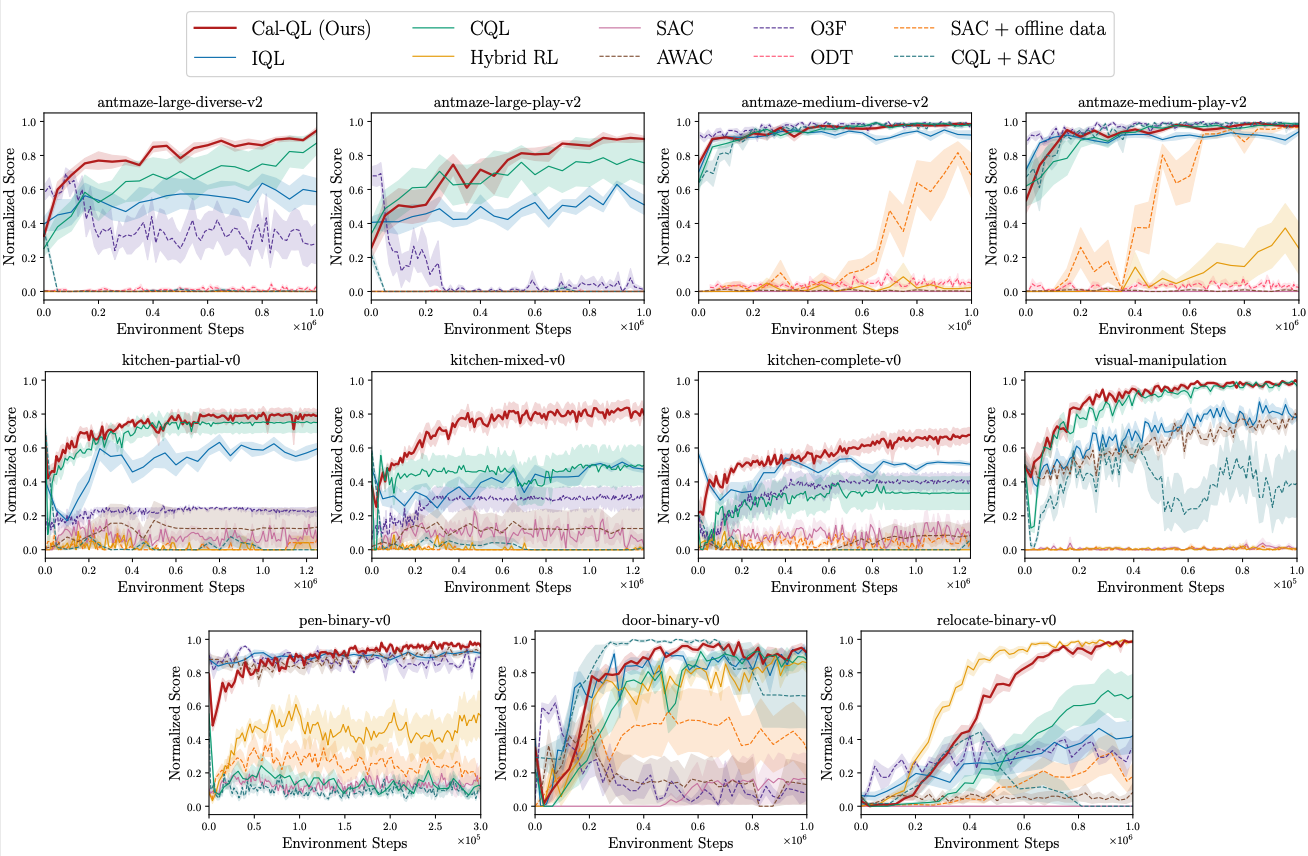Figure 6: Comparison of the results of online fine tuning, starting with the measures pre-trained by each method as initial measures.

The above figure compares the performance of each benchmark during fine tuning. It can be seen that Cal-QL improves the convergence speed and final performance during online fine tuning for many tasks.

In order to prevent "unlearn" completely, it is necessary to calibrate all the suboptimal measures, but in the above experiment, only the behavioral measures are calibrated, and it can be seen that this alone stabilizes and improves the efficiency of learning. They also confirm that there is no significant performance degradation when the values are estimated by neural networks instead of empirical averages, and that the performance is not sensitive to the estimation error of the value function of the reference measure.

## Summary

In this issue, we focus on off-line reinforcement learning as a pre-training method, and introduce a paper proposing an off-line reinforcement learning method that enables efficient fine tuning. The idea is supported by experiments, the implementation is simple, and the effectiveness is experimentally verified. Off-line reinforcement learning + on-line fine tuning is a hot topic with high practical applicability, and is being studied day by day. Please keep an eye on these keywords.

If you have any suggestions for improvement of the content of the article,